Advertisement Remove all ads

# Engineering Mechanics CBCGS 2017-2018 BE Computer Engineering Semester 1 (FE First Year) Question Paper Solution

Advertisement Remove all ads
Engineering Mechanics [CBCGS]
Marks: 80Academic Year: 2017-2018
Date: December 2017

1 | Attempt any four questions
1.a

state and prove varigon’s theorem.

Concept: Varignon’s Theorem
Chapter:  System of Coplanar Forces
1.b

Find the resultant of the force system as shown in the given figure.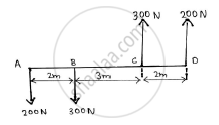Concept: Resultant of concurrent forces
Chapter:  System of Coplanar Forces
1.c

Find the co-ordinate of the centroid of the area as shown in the given figure.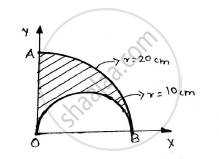Concept: Velocity and Acceleration in Terms of Rectangular Co-ordinate System
Chapter:  Kinematics of Particle
1.d

A force of 500 N is acting on a block of 50 kg mass resting on a horizontal surface as shown in the figure. Determine the velocity after the block has travelled a distance of 10m. Co efficient of kinetic friction is 0.5.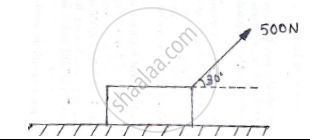Concept: Cone of Friction
Chapter:  Friction
1.e

The position vector of a particle which moves in the X-Y plane is given by 𝒓̅ = (3t3-4t2)𝒊̅ + (0.5t4)𝒋̅

Concept: Velocity and Acceleration in Terms of Rectangular Co-ordinate System
Chapter:  Kinematics of Particle
2
2.a

Find the resultant of the force acting on the bell crank lever shown. Also locate its position with respect to hinge B.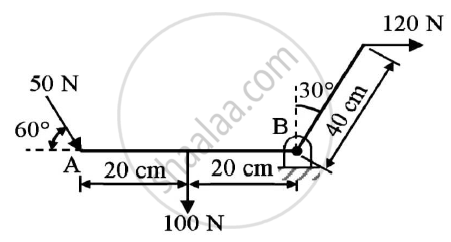Given : Forces on the bell crank lever
To find : Resultant and it’s position w.r.t hinge B

Concept: Resultant of parallel forces
Chapter:  System of Coplanar Forces
2.b

Determine the reaction at points of constant 1,2 and 3. Assume smooth surfaces.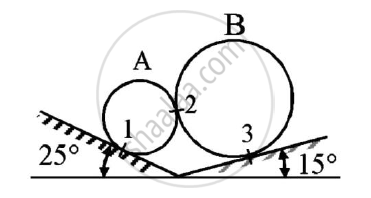Given: The spheres are in equilibrium
To find: Reactions at points 1,2 and 3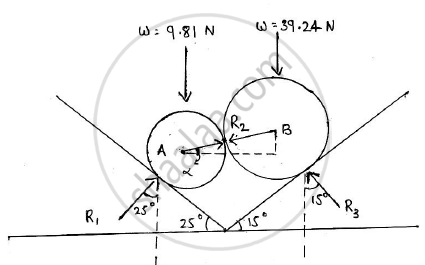Concept: Condition of Equilibrium for non-concurrent nonparallel general forces
Chapter:  Equilibrium of System of Coplanar Forces
Advertisement Remove all ads
2.c

Two balls having 20kg and 30 kg masses are moving towards each other with velocities of 10 m/s and 5 m/s respectively as shown in the figure.
If after the impact ,the ball having 30 kg mass is moving with 6 m/s velocity to the right then determine the coefficient of restitution between the two balls.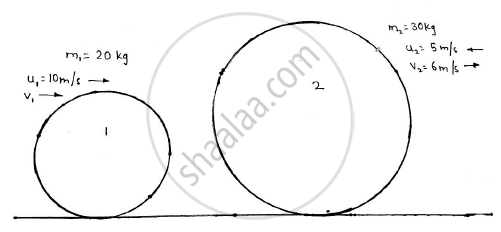Concept: Velocity and Acceleration in Terms of Rectangular Co-ordinate System
Chapter:  Kinematics of Particle
3
3.a

Determine the position of the centroid of the plane lamina. Shaded portion is removed.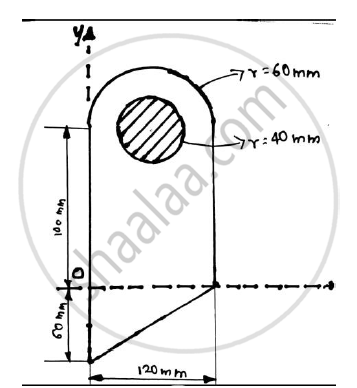Concept: Centroid for Plane Laminas
Chapter:  Center of Gravity and Centroid for Plane Laminas
3.b

Explain the conditions for equilibrium of forces in space.

Concept: Condition of equilibrium for concurrent forces
Chapter:  Equilibrium of System of Coplanar Forces
3.c

A 30 kg block is released from rest.If it slides down from a rough incline which is having co-efficient of friction 0.25.Determine the maximum compression of the spring.Take k=1000 N/m.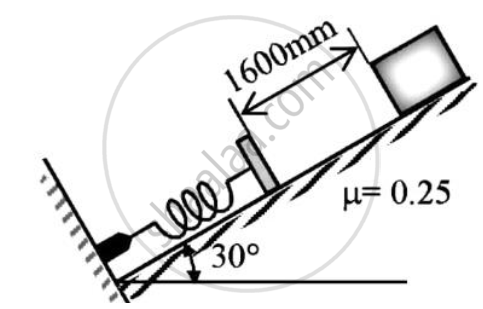Concept: Equilibrium of Bodies on Inclined Plane
Chapter:  Friction
4
4.a

Find the support reactions at A and B for the beam loaded as shown in the given figure.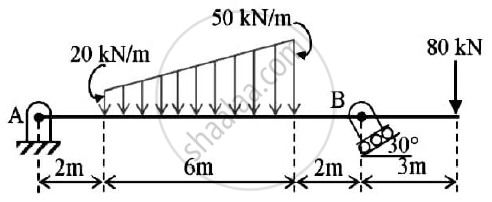Concept: Condition of equilibrium for parallel forces
Chapter:  Equilibrium of System of Coplanar Forces
4.b

The V-X graph of a rectilinear moving particle is shown. Find the acceleration of the particle at 20m,80 m and 200 m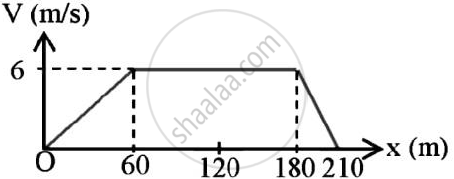Concept: Velocity and Acceleration in Terms of Rectangular Co-ordinate System
Chapter:  Kinematics of Particle
4.c

A bar 2 m long slides down the plane as shown.The end A slides on the horizontal floor with a velocity of 3 m/s.Determine the angular velocity of rod AB and the velocity of end B for the position shown.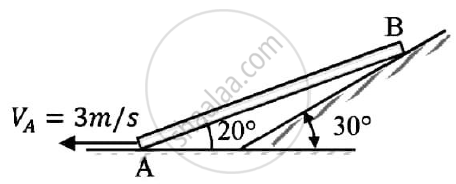Concept: Velocity and Acceleration in Terms of Rectangular Co-ordinate System
Chapter:  Kinematics of Particle
Advertisement Remove all ads
5
5.a

Referring to the truss shown in the figure. Find :
(a) Reaction at D and C
(b)Zero force members.
(c)Forces in member FE and DC by method of section.
(d)Forces in other members by method of joints.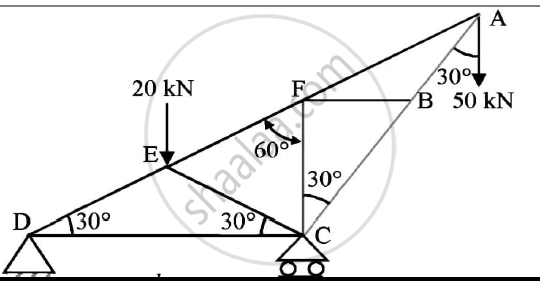Concept: Analysis of Plane Trusses by Using Method of Joints
Chapter:  Analysis of Plane Trusses
5.b

Determine the force P required to move the block A of 5000 N weight up the inclined plane, coefficient of friction between all contact surfaces is 0.25. Neglect the weight of the wedge and the wedge angle is 15 degrees.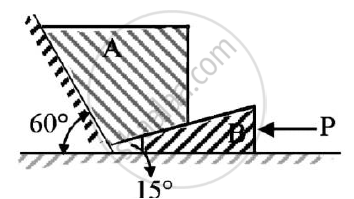Given : Weight of block A = 5000 N
μs=0.25
Wedge angle = 15º

To find : Force P required to move block A up the inclined plane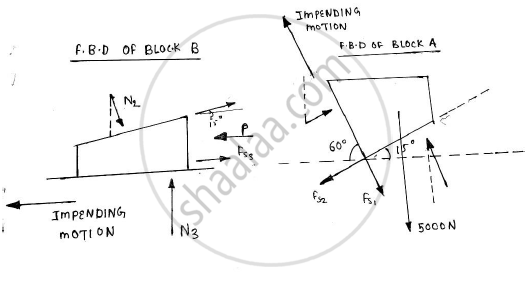Concept: Condition of equilibrium for concurrent forces
Chapter:  Equilibrium of System of Coplanar Forces
5.c

Determine the tension in a cable BC shown in fig by virtual work method.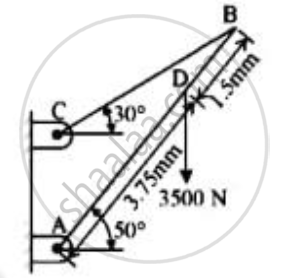Given: F=3500 N
ϴ = 50o
Length of rod = 3.75 mm + 1.5 mm = 5.25 mm
To find : Tension in cable BC

Concept: Applications on Equilibrium Mechanisms
Chapter:  Principle of Virtual Work
6
6.a

A 500 N Crate kept on the top of a 15° sloping surface is pushed down the plane with an initial velocity of 20m/s. If μs = 0.5 and μk = 0.4, determine the distance travelled by the block and the time it will take as it comes to rest.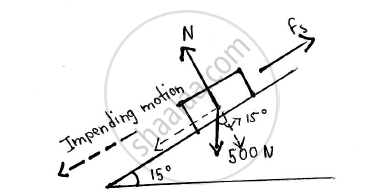Given: Weight of crate = 500 N

Initial velocity(u) = 20 m/s

μs = 0.5

μk = 0.4

θ = 15°

Final velocity (v) = 0 m/s
To find: Distance travelled by the block Time it will take before coming to rest

Concept: Velocity and Acceleration in Terms of Rectangular Co-ordinate System
Chapter:  Kinematics of Particle
6.b

Derive the equation of path of a projectile and hence show that equation of path of projectile is a parabolic curve.

Concept: Velocity and Acceleration in Terms of Rectangular Co-ordinate System
Chapter:  Kinematics of Particle
6.c

particle is moving in X-Y plane and it’s position is defined bybar r=(3/2 t^2)bar l+(2/3 t^3) barJ "Find radius of curvature when t=2sec."

Concept: Velocity and Acceleration in Terms of Rectangular Co-ordinate System
Chapter:  Kinematics of Particle
6.d

A Force of 100 N acts at a point P(-2,3,5)m has its line of action passing through Q(10,3,4)m. Calculate moment of this force about origin (0,0,0).

Concept: Velocity and Acceleration in Terms of Rectangular Co-ordinate System
Chapter:  Kinematics of Particle
Advertisement Remove all ads

#### Question PapersVIEW ALL 

Advertisement Remove all ads

#### Request Question Paper

If you dont find a question paper, kindly write to us

View All Requests

#### Submit Question Paper

Help us maintain new question papers on Shaalaa.com, so we can continue to help students

only jpg, png and pdf files

## University of Mumbai previous year question papers Semester 1 (FE First Year) Engineering Mechanics with solutions 2017 - 2018

University of Mumbai Semester 1 (FE First Year) Engineering Mechanics question paper solution is key to score more marks in final exams. Students who have used our past year paper solution have significantly improved in speed and boosted their confidence to solve any question in the examination. Our University of Mumbai Semester 1 (FE First Year) Engineering Mechanics question paper 2018 serve as a catalyst to prepare for your Engineering Mechanics board examination.
Previous year Question paper for University of Mumbai Semester 1 (FE First Year) Engineering Mechanics-2018 is solved by experts. Solved question papers gives you the chance to check yourself after your mock test.
By referring the question paper Solutions for Engineering Mechanics, you can scale your preparation level and work on your weak areas. It will also help the candidates in developing the time-management skills. Practice makes perfect, and there is no better way to practice than to attempt previous year question paper solutions of University of Mumbai Semester 1 (FE First Year).

How University of Mumbai Semester 1 (FE First Year) Question Paper solutions Help Students ?
• Question paper solutions for Engineering Mechanics will helps students to prepare for exam.
• Question paper with answer will boost students confidence in exam time and also give you an idea About the important questions and topics to be prepared for the board exam.
• For finding solution of question papers no need to refer so multiple sources like textbook or guides.
Advertisement Remove all ads
Share
Notifications

View all notifications

Forgot password?